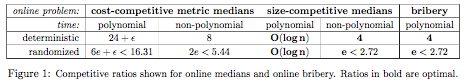## neal young / Chrobak08Online

•Following Mettu and Plaxton, we study incremental algorithms for the $$k$$-medians problem. Such an algorithm must produce a nested sequence $$F_1 \subseteq F_2 \subseteq \cdots \subseteq F_n$$ of sets of facilities. Mettu and Plaxton show that incremental metric medians has a (roughly) $$40$$-competitive deterministic polynomial-time algorithm. We give improved algorithms, including a $$(24+\epsilon)$$-competitive deterministic polynomial-time algorithm and a $$5.44$$-competitive, randomized, non-polynomial-time algorithm.

We also consider the competitive ratio with respect to size. An algorithm is $$s$$-size-competitive if, for each $$k$$, the cost of $$F_k$$ is at most the minimum cost of any set of $$k$$ facilities, while the size of $$F_k$$ is at most $$s k$$. We present optimally competitive algorithms for this problem.

Our proofs reduce incremental medians to the following online bidding problem: faced with some unknown threshold $$T>0$$, an algorithm must submit bids'' $$b>0$$ until it submits a bid as large as $$T$$. The algorithm pays the sum of its bids. We describe optimally competitive algorithms for online bidding.

Our results on cost-competitive incremental medians extend to approximately metric distance functions, incremental fractional medians, and incremental bicriteria approximation.
Journal version of .